# HSSlive: Plus One & Plus Two Notes & Solutions for Kerala State Board

## BSEB Class 10 Maths Chapter 1 Real Numbers Ex 1.4 Textbook Solutions PDF: Download Bihar Board STD 10th Maths Chapter 1 Real Numbers Ex 1.4 Book AnswersBSEB Class 10 Maths Chapter 1 Real Numbers Ex 1.4 Textbook Solutions PDF: Download Bihar Board STD 10th Maths Chapter 1 Real Numbers Ex 1.4 Book Answers

BSEB Class 10th Maths Chapter 1 Real Numbers Ex 1.4 Textbooks Solutions and answers for students are now available in pdf format. Bihar Board Class 10th Maths Chapter 1 Real Numbers Ex 1.4 Book answers and solutions are one of the most important study materials for any student. The Bihar Board Class 10th Maths Chapter 1 Real Numbers Ex 1.4 books are published by the Bihar Board Publishers. These Bihar Board Class 10th Maths Chapter 1 Real Numbers Ex 1.4 textbooks are prepared by a group of expert faculty members. Students can download these BSEB STD 10th Maths Chapter 1 Real Numbers Ex 1.4 book solutions pdf online from this page.

## Bihar Board Class 10th Maths Chapter 1 Real Numbers Ex 1.4 Books Solutions

 Board BSEB Materials Textbook Solutions/Guide Format DOC/PDF Class 10th Subject Maths Chapter 1 Real Numbers Ex 1.4 Chapters All Provider Hsslive

## How to download Bihar Board Class 10th Maths Chapter 1 Real Numbers Ex 1.4 Textbook Solutions Answers PDF Online?

2. Click on the Bihar Board Class 10th Maths Chapter 1 Real Numbers Ex 1.4 Answers.
3. Look for your Bihar Board STD 10th Maths Chapter 1 Real Numbers Ex 1.4 Textbooks PDF.
4. Now download or read the Bihar Board Class 10th Maths Chapter 1 Real Numbers Ex 1.4 Textbook Solutions for PDF Free.

## BSEB Class 10th Maths Chapter 1 Real Numbers Ex 1.4 Textbooks Solutions with Answer PDF Download

Find below the list of all BSEB Class 10th Maths Chapter 1 Real Numbers Ex 1.4 Textbook Solutions for PDF’s for you to download and prepare for the upcoming exams:

## BSEB Bihar Board Class 10th Maths Solutions Chapter 1 Real Numbers Ex 1.4

Question 1.
Without actually performing the long division, state whether the following rational numbers will have a terminating decimal expansion or a non-terminating repeating decimal expansion:

1. 133125
2. 178
3. 64455
4. 151600
5. 29343
6. 232352
7. 129225775
8. 615
9. 3550
10. 77210

Solution:
We know that if the denominator of a rational number has no prime factors other than 2 or 5 (or both), then it is expressible as a terminating decimal, otherwise it has non-terminating repeating decimal expansion.
Thus, we will have to check the prime factors of the denominators of each of the given rational numbers.

1. In, the denominator is 3125.53125
We have: 3125 = 5 × 5 × 5 × 5 ×
Thus, 3125 has 5 as the only prime factor.
Hence, 133125 must have a terminating decimal expansion.2. In the denominator is 8.
We have: 8 = 2 × 2 × 2
Thus, 8 has 2 as the only prime factor.
Hence, 178 must have a terminating decimal expansion.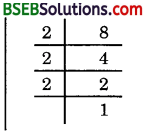3. In 64455, denominator is 455.
We have: 455 = 5 × 7 × 13
Clearly, 455 has prime factors ther than 2 and 5. So, it will not have a terminating decimal expansion.
That is, it will have non-terminating repeating decimal expansion.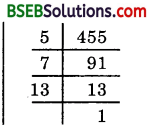4. In 151600, the denominator is 1600.
We have:
1600 = 2 × 2 × 2 × 2 × 2 × 2 × 5 × 5
Thus, 1600 has only 2 and 5 as prime factors.
Hence, 151600 must have a terminating decimal expansion.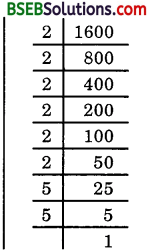5. In 29343, the denominator is 343.
We have:
343 = 7 × 7 × 7
Clearly, 343 has prime factors other than 2 and 5.
So, it will have non-terminating repeating decimal expansion.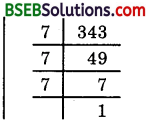6. In 2323⋅52
Clearly, the denominator 23.52 has only 2 and 5 as prime factors.
Hence, 2323⋅52 must have a terminating decimal expansion.

7. In 12922⋅57⋅75
Clearly, the denominator 23.57.75 has prime factors other than 2 and 5. So, it will have non-terminating repeating decimal expansion.

8. In 615, we have 615 = 25
Clearly, denominator 5 has no prime factors other than 2 and 5. So, it will have a terminating decimal expansion.

9. in 3550, we have denominator 50 = 2 × 5 × 5
The denominator has only 2 and 5 as prime factors.
Hence, 3550 must have a terminating decimal expansion.

10. In 77210, we have 77210 = 1130. The denominator is 30.
We have: 30 = 2 × 3 × 5
Clearly, 30 has prime factors other than 2 and 5.
So, it will have non-terminating repeating decimal expansion.Question 2.
Write down the decimal expansion of those rational numbers in Question 1 above which have terminating decimal expansions.
Solution:
1.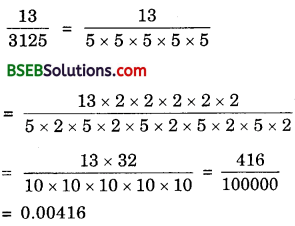2.3. Non-terminating repeating.

4.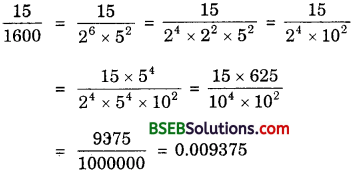5. Non-terminating repeating.

6.7. Non-terminating repeating.

8. 615 = 25
= 410 = 0.4

9. 3550 = 35×250×2 = 70100 = 0.70

10. Non-terminating repeating.

Question 3.
The following real numbers have decimal expansions as given below. In each case, decide whether they are rational or not. If they are rational, and of the form 𝑝𝑞, what can you say about the prime factors of q?

1. 43.123456789
2. 0.120120012000120000…
3. 43.123456789⎯⎯⎯⎯⎯⎯⎯⎯⎯⎯⎯⎯⎯⎯⎯⎯⎯⎯⎯⎯⎯⎯⎯⎯⎯⎯

Solution:
1. 43.123456789 is terminating.
So, it represents a rational number.
Thus, 43.123456789 = 431234567891000000000 = 𝑝𝑞. Thus, q = 109.

2. 0.12012001200012000… is non-terminating and non-repeating. So, it is irrational.

3. 43.123456789⎯⎯⎯⎯⎯⎯⎯⎯⎯⎯⎯⎯⎯⎯⎯⎯⎯⎯⎯⎯⎯⎯⎯⎯⎯⎯ is non-terminating but repeating. So, it is rational.
Thus, 43.123456789⎯⎯⎯⎯⎯⎯⎯⎯⎯⎯⎯⎯⎯⎯⎯⎯⎯⎯⎯⎯⎯⎯⎯⎯⎯⎯ = 431234564699999999 = 𝑝𝑞.
Thus, q = 999999999.

## Bihar Board Class 10th Maths Chapter 1 Real Numbers Ex 1.4 Textbooks for Exam Preparations

Bihar Board Class 10th Maths Chapter 1 Real Numbers Ex 1.4 Textbook Solutions can be of great help in your Bihar Board Class 10th Maths Chapter 1 Real Numbers Ex 1.4 exam preparation. The BSEB STD 10th Maths Chapter 1 Real Numbers Ex 1.4 Textbooks study material, used with the English medium textbooks, can help you complete the entire Class 10th Maths Chapter 1 Real Numbers Ex 1.4 Books State Board syllabus with maximum efficiency.

## FAQs Regarding Bihar Board Class 10th Maths Chapter 1 Real Numbers Ex 1.4 Textbook Solutions

#### Can we get a Bihar Board Book PDF for all Classes?

Yes you can get Bihar Board Text Book PDF for all classes using the links provided in the above article.

## Important Terms

Bihar Board Class 10th Maths Chapter 1 Real Numbers Ex 1.4, BSEB Class 10th Maths Chapter 1 Real Numbers Ex 1.4 Textbooks, Bihar Board Class 10th Maths Chapter 1 Real Numbers Ex 1.4, Bihar Board Class 10th Maths Chapter 1 Real Numbers Ex 1.4 Textbook solutions, BSEB Class 10th Maths Chapter 1 Real Numbers Ex 1.4 Textbooks Solutions, Bihar Board STD 10th Maths Chapter 1 Real Numbers Ex 1.4, BSEB STD 10th Maths Chapter 1 Real Numbers Ex 1.4 Textbooks, Bihar Board STD 10th Maths Chapter 1 Real Numbers Ex 1.4, Bihar Board STD 10th Maths Chapter 1 Real Numbers Ex 1.4 Textbook solutions, BSEB STD 10th Maths Chapter 1 Real Numbers Ex 1.4 Textbooks Solutions,
Share: